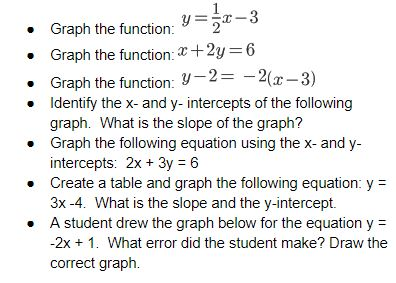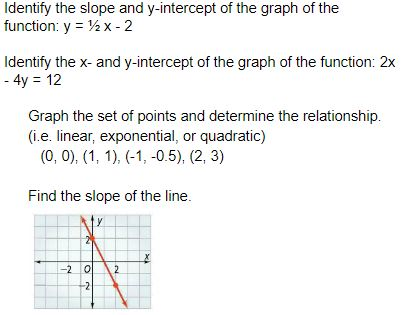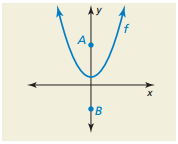#### MAT-HS.F-IF.07.a

 MAT-HS Targeted Standards(F) Concept: Function(IF) Domain: Interpreting FunctionsCluster: Analyze functions using different representations MAT-HS.F-IF.07* Graph functions expressed symbolically and show key features of the graph, by hand in simple cases and using technology for more complicated cases. Graph linear functions and show intercepts, maxima, and minima where appropriate.

## Student Learning Targets:

• I can
• I can

• I can
• I can

### Skills (Performance) Targets

• I can graph a linear equation and show intercepts. (HS.F-IF.7.a)

• I can
• I can

## Proficiency Scale

 Score Description Sample Activity 4.0 (advanced) In addition to Score 3.0, the student demonstrates in-depth inferences and applications regarding more complex material that go beyond end of instruction expectations. -Student is able to determine and describe intervals where the function is increasing and decreasing using interval notation. 3.5 In addition to Score 3.0 performance, the student demonstrates in-depth inferences and applications regarding the more complex content with partial success. 3.0 (proficient) The student can: interpret and sketch key features of the linear graph (HS.F-IF.7a1) including: intercepts positive/negative slope construct simple graphs by hand and use technology for more complicated cases. The student exhibits no major errors or omissions.2.5 The student demonstrates no major errors or omissions regarding the simpler details and processes (Score 2.0 content) and partial knowledge of the more complex ideas and processes (Score 3.0 content). 2.0 (progressing) There are no major errors or omissions regarding the simpler details and processes as the student can: recognize and recall basic terminology, such as: linear equation. quadratic equation. exponential equation. slope intercepts only interprets and sketches some of the key features of the graph. can identify if a function is linear, exponential, or quadratic However, the student exhibits major errors or omissions regarding the more complex ideas and processes.1.5 The student demonstrates partial knowledge of the simpler details and processes (Score 2.0 content) but exhibits major errors or omissions regarding the more complex ideas and procedures (Score 3.0 content). 1.0 (beginning) With help, the student demonstrates a partial understanding of some of the simpler details and processes (Score 2.0 content) and some of the more complex ideas and processes (Score 3.0 content). - 0.5 With help, the student demonstrates a partial understanding of some of the simpler details and processes (Score 2.0 content) but not the more complex ideas and processes (Score 3.0 content). 0.0 Even with help, the student demonstrates no understanding or skill. -

## Alg II Graph Quadratic Functions Proficiency Scale

 Score Description Sample Activity 4.0 (advanced) In addition to Score 3.0, the student demonstrates in-depth inferences and applications regarding more complex material that go beyond end of instruction expectations. The graph of  f(x)=ax2+c is shown Points A and B are the same distance from the vertex of the graph of f.  Which point is closer to the vertex of the graph of  f as c increases? Explain your reasoning.3.5 In addition to Score 3.0 performance, the student demonstrates in-depth inferences and applications regarding the more complex content with partial success. 3.0 (proficient) The student can: graph quadratic functions in any form expressed symbolically and show key features of the graph (graph simple cases by hand and use technology to show more complicated cases). The student exhibits no major errors or omissions. Graph the following equations and identify all the key features including:  vertex, x-intercepts, y-intercepts, increasing interval, decreasing interval, maximums, minimums, axis of symmetry,  end behaviors, domain and range.2.5 The student demonstrates no major errors or omissions regarding the simpler details and processes (Score 2.0 content) and partial knowledge of the more complex ideas and processes (Score 3.0 content). 2.0 (progressing) There are no major errors or omissions regarding the simpler details and processes as the student can: recognize and recall basic terminology, such as: quadratic function vertex x- intercepts y- intercepts zeros solutions roots intervals minimum/maximum values axis of symmetry domain range However, the student exhibits major errors or omissions regarding the more complex ideas and processes. Given a graph,  identify all the key features including: vertex,x-intercepts, y-intercepts, increasing interval, decreasing interval, maximums, minimums, axis of symmetry, end behaviors, domain and range. 1.5 The student demonstrates partial knowledge of the simpler details and processes (Score 2.0 content) but exhibits major errors or omissions regarding the more complex ideas and procedures (Score 3.0 content). 1.0 (beginning) With help, the student demonstrates a partial understanding of some of the simpler details and processes (Score 2.0 content) and some of the more complex ideas and processes (Score 3.0 content). 0.5 With help, the student demonstrates a partial understanding of some of the simpler details and processes (Score 2.0 content) but not the more complex ideas and processes (Score 3.0 content). 0.0 Even with help, the student demonstrates no understanding or skill.

## Resources

 Web Vocab intercepts maximum minimum square root function cube root function piecewise-defined function step function absolute value function polynomial function zeroes exponential function